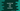# JavaScript program to add two numbers - 3 different ways## JavaScript program to add two numbers:

This is a beginner level JavaScript question. With this program, you will learn how to do mathematical calculations in JavaScript. This problem can be solved in many ways. In this post, I will show you three different ways to write a program that adds two numbers in JavaScript.

### Add two numbers in JavaScript, the basic way:

This is the basic way to add numbers in JavaScript. We will define two const values holding two numbers and add them using +.

Below is the complete program:

``````const firstNumber = 1
const secondNumber = 2

console.log(firstNumber + secondNumber)``````

If you run it, it will print 3 as output.

Here, We defined two const values firstNumber and secondNumber. firstNumber is equal to 1 and secondNumber is equal to 2 The log statement is printing the sum of these two values.

### Method 2: By taking the numbers as user input:

We can also read the numbers as user input and find out the sum as like below:

``````const firstNumber = parseInt(prompt("Enter the first number : "))
const secondNumber = parseInt(prompt("Enter the second number : "))

console.log(firstNumber + secondNumber)``````

This program will work only in a Browser. If you are using Google Chrome or any Chromium based browser, right click on a web page, click on inspect, move to the console tab and paste the above program. It will show you one prompt to enter the first and second numbers. Then, it will print the sum of the numbers you entered.

### Method 3: HTML and JavaScript:

We can write one HTML file and take the user inputs in two input boxes. You can open the .html file in a web browser and verify it. Create one .html file with the below content:

``````<!DOCTYPE html>
<html lang="en">
<script>
var first, second;

first = Number(document.getElementById("firstInput").value);
second = Number(document.getElementById("secondInput").value);

document.getElementById("result").value = first + second;
}
</script>